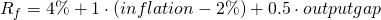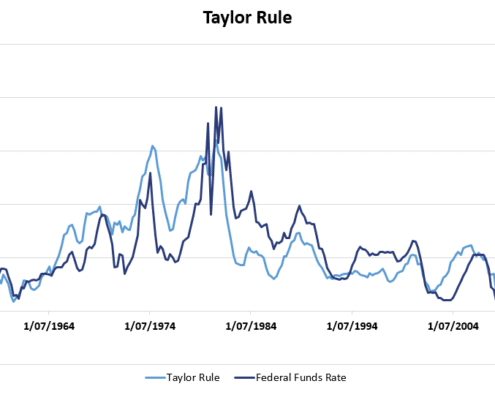# Taylor Rule

The Taylor Rule is a monetary policy rule in economics. The rule is called the Taylor Rule because it was proposed by John B. Taylor in 1993. It describes a central bank’s monetary policy when the bank determines its monetary policy based on price stability and economic output.

## Taylor Rule definition

The Taylor rule is based on the observation that, in the United States at least, the central bank has a “dual mandate”. In particular, the Federal Reserve (FED) tries to maintain price stability and maximum employment. Such a mutual mandate can be summarized using the Taylor Rule for monetary policy. The rule states that the nominal interest rate (Rf) can be approximated as follows:where the output gap is typically defined as the “percentage deviation of real GDP from its target”. The output gap measures whether output is above or below its ‘potential’.

## Taylor rule formula interpretation

The Taylor rule formula above clearly shows that nominal interest rate is determined both by inflation (price stability) and output gat (employment and growth). The Taylor rule formula therfore clearly reflects the dual mandate of the Fed.

To see this, let’s first assume that inflation is at 2% and that the output gap is at zero. In that case,  the Fed is expected to set a nominal interest rate of 4%. Given an inflation rate of 2%, this corresponds to a a real interest rate (Rf—inflation) of 2%.

Next, imagine the inflation rate rises above 2%. In that case, the Fed is expected to raise nominal interest rates more than one-for-one. This is called the the “Taylor principle”. In particular, if inflation rate goes up to 3%, then the Fed is expected to increase the nominal rate to 5.5%. The real interest rate goes up to 2.5%. This exceeds the inflation rate and is meant to cool the economy. This should bring the inflation back down toward its target. Similarly, a negative output gap, i.e., high unemployment, results in lower interest rates that stimulate the economy.

Below, we plot a Taylor rule graph for the the Fed. The graph shows the expected interest rate based on the inflation rate and the output gap for the US.The data used to construct this figure is provided by the FRED and can be downloaded here.

The Taylor rule is, of course, only an approximation. It also only describes the possible behavior of central banks that have a dual mandate. The European Central Bank (ECB) for example only targets price stability and does not consider the output gap when setting the short term interest rate.

## Summary

We discussed a very simple formula that can be used to model a central bank’s monetary policy decisions. This rule, called the Taylor rule, worked well historically. Since the financial crisis, however, the rule no longer fits monetary policy that well.

### Taylor rule calculator

The following Excel file implements a Taylor Rule calculator: